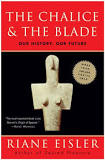# Astrological Trine Stems From the 3 x 3 Number Square

Astrological Trine Stems From the 3 x 3 Number Square. The astrological aspects are noted in the central circle of this natal chart, where the different colors and symbols distinguish between the different aspects, such as the square (red) or trine (green).

### Trine

A trine (abbrev. Tri) is an angle of 120° (1/3 of the 360° ecliptic), an orb of somewhere between 5° and 10° . The trine indicates harmony and ease. The trine is a source of artistic and creative talent, which is innate. The trine has been traditionally assumed to be extremely beneficial.

### How Does the Astrological Trine Tie into the 3 x 3 Number Square?Answer, in almost every conceivable way. The single core number the above square is 5. Consider the following:

1. The trine has an angle of 120°. Multiply the numbers 1  thru 5. Thus, 1 x 2 x 3 x 4 x 5 = 120. You have your trine by number.
2. Now, add the same numbers 1-5. Thus, 1 + 2 + 3 + 4 + 5 = 15.  We have the following congruence with this sum.  Any row of three numbers on this square totals 15. For example, added horizontally we see that 4 + 9 + 2 = 15.  Vertically we get the same totals as 4 + 3 + 8 = 15.  Diagonally, likewise the same. 4 + 5 + 6 = 15. You can find fifteen in eight different ways on this square: Three diagonally, three vertically and  two horizontally. We now have 8 x 15 = 120. Here is yet another congruence with the 120 astrological trine number.
3. As you already have read above, the elliptic orb is somewhere between the boundaries of 5° and  10°. We have the following on the square of thee with the numbers of these boundaries: The central number is five. Any two opposite numbers are 10. An example being (4 + 6)  or ( 9 + 1).Wheel of the zodiac: This 6th century mosaic pavement in a synagogue incorporates Greek-Byzantine elements, Beit Alpha, Israel.

At one time knowledge was based on number squares. This includes the knowledge of the sciences and arts of mankind. As mentioned, the trine has been traditionally assumed to be extremely beneficial. It too, is associated with a number square, being 3 x 3. I say, to understand a more peaceful past, at which time the cycle of yin dominated the world, study number squares. It seems that most of this knowledge was destroyed when the Library at Alexandria, Egypt was burned down. My work on number squares has been self-directed. However, my source for the existence of more peaceful times is Riane Eisler  who wrote, The Chalice and the Blade. Her book is well worth reading. Riane Eisler. Riane Tennenhaus Eisler (born July 22, 1931) is a cultural historian, systems scientist, educator, attorney, speaker, and author whose work on cultural transformation has inspired scholars.